### Electrostatics

The governing equations of electrostatics are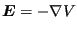(369)

and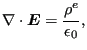(370)

where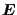is the electric field,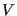is the electric potential,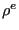is the electric charge density and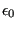is the permittivity of free space (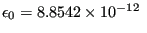C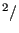Nm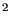). The electric fieldis the force on a unit charge.

In metals, it is linked to the current density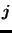by the electric conductivity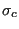: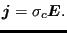(371)

In free space, the electric field is locally orthogonal to a conducting surface. Near the surface the size of the electric field is proportional to the surface charge density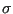: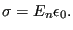(372)

Substituting Equation (369) into Equation (370) yields the governing equation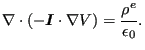(373)

Accordingly, by comparison with the heat equation, the correspondence in Table  (13) arises. Notice that the electrostatics equation is a steady state equation, and there is no equivalent to the heat capacity term.

 heat electrostatics T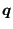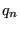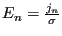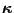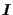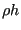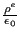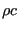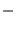An application of electrostatics is the potential drop technique for crack propagation measurements: a predefined current is sent through a conducting specimen. Due to crack propagation the specimen section is reduced and its electric resistance increases. This leads to an increase of the electric potential across the specimen. A finite element calculation for the specimen (electrostatic equation with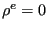) can determine the relationship between the potential and the crack length. This calibration curve can be used to derive the actual crack length from potential measurements during the test.

Another application is the calculation of the capacitance of a capacitor. Assuming the space within the capacitor to be filled with air, the electrostatic equation withapplies (since there is no charge within the capacitor). Fixing the electric potential on each side of the capacitor (to e.g. zero and one), the electric field can be calculated by the thermal analogy. This field leads to a surface charge density by Equation (372). Integrating this surface charge leads to the total charge. The capacitance is defined as this total charge divided by the electric potential difference (one in our equation).

For dielectric applications Equation (370) is modified into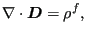(374)

where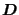is the electric displacement and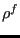is the free charge density . The electric displacement is coupled with the electric field by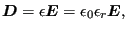(375)

where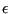is the permittivity and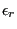is the relative permittivity (usually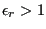, e.g. for silicon=11.68). Now, the governing equation yields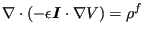(376)

and the analogy in Table (14) arises. The equivalent of Equation (372) now reads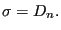(377)

 heat electrostatics T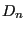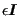The thermal equivalent of the total charge on a conductor is the total heat flow. Notice thatmay be a second-order tensor for anisotropic materials.